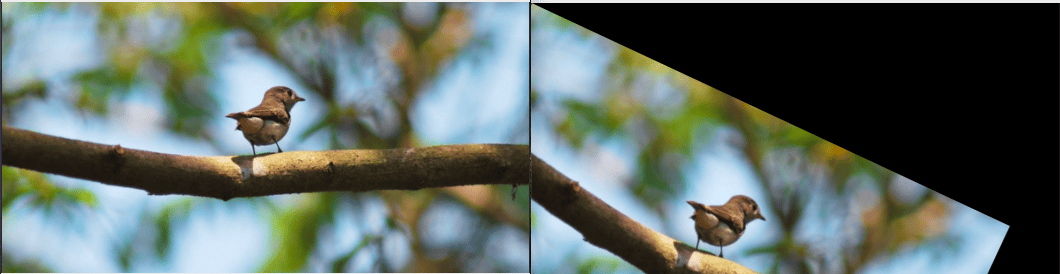# How to Rotate an Image in Python using OpenCV

Image rotation is a specialization of an affine transformation. The OpenCV-Python module deals with real-time applications related to computer vision. It provides several inbuilt functions to deal with images as input from the user. We use cv2.getRotationMatrix2D() method to transform the matrix and cv2.warpAffine() method to rotate the image based on the matrix transformation.

## Rotate Image in Python using OpenCV

To rotate an image, apply a matrix transformation.  To create a matrix transformation, use the cv2.getRotationMatrix2D() method and pass the origin that we want the rotation to happen around. If we pass the origin (0, 0), then it will start transforming the matrix from the top-left corner. The getRotationMatrix2D() function calculates the affine matrix of 2D rotation.

Then we can specify the degrees by which we want the rotation to occur. For example, -25 degrees, then we can pass in a value of one.

In the last step,  use this transformation matrix to rotate an image using the cv2.warpAffine() method, and then we need to pass in the shape of our image.

The warpAffine() function applies an affine transformation to the image. It transforms the source image using the specified matrix.

```# app.py

import numpy as np
import cv2

cv2.imshow('Original', img)

M = cv2.getRotationMatrix2D((0, 0), -25, 1)
rotated = cv2.warpAffine(img, M, (img.shape, img.shape))

cv2.imshow('Rotated Image', rotated)

cv2.waitKey(0)
cv2.destroyAllWindows()```

#### OutputFrom the output, you can see that our image is rotated from the upper left corner to -25 degrees. Image rotation is the geometric transformation and it can be done via either forward mapping or inverse mapping.

Let’s rotate the image from the origin (150, 150) to -30 degree.

```# app.py

import numpy as np
import cv2

cv2.imshow('Original', img)

M = cv2.getRotationMatrix2D((150, 150), -30, 1)
rotated = cv2.warpAffine(img, M, (img.shape, img.shape))

cv2.imshow('Rotated Image', rotated)

cv2.waitKey(0)
cv2.destroyAllWindows()```

#### OutputIn Affine transformation, all parallel lines of an original image will still be similar in the output image.

## Conclusion

The warpAffine() and getRotationMatrix2D() methods perform the various geometrical transformations of 2D images depending on the what arguments you will provide. These functions do not modify the original image content but deform the pixel grid and map this deformed grid to the final rotated image.

That is it to rotate an image in Python using OpenCV.

This site uses Akismet to reduce spam. Learn how your comment data is processed.